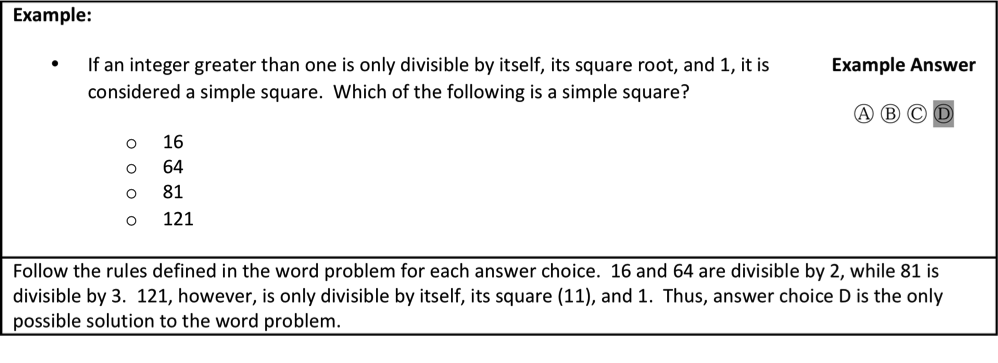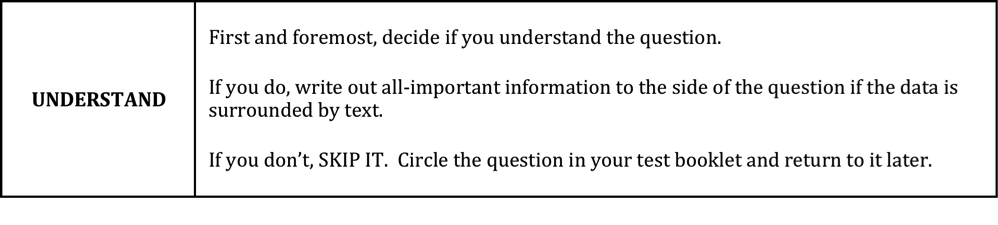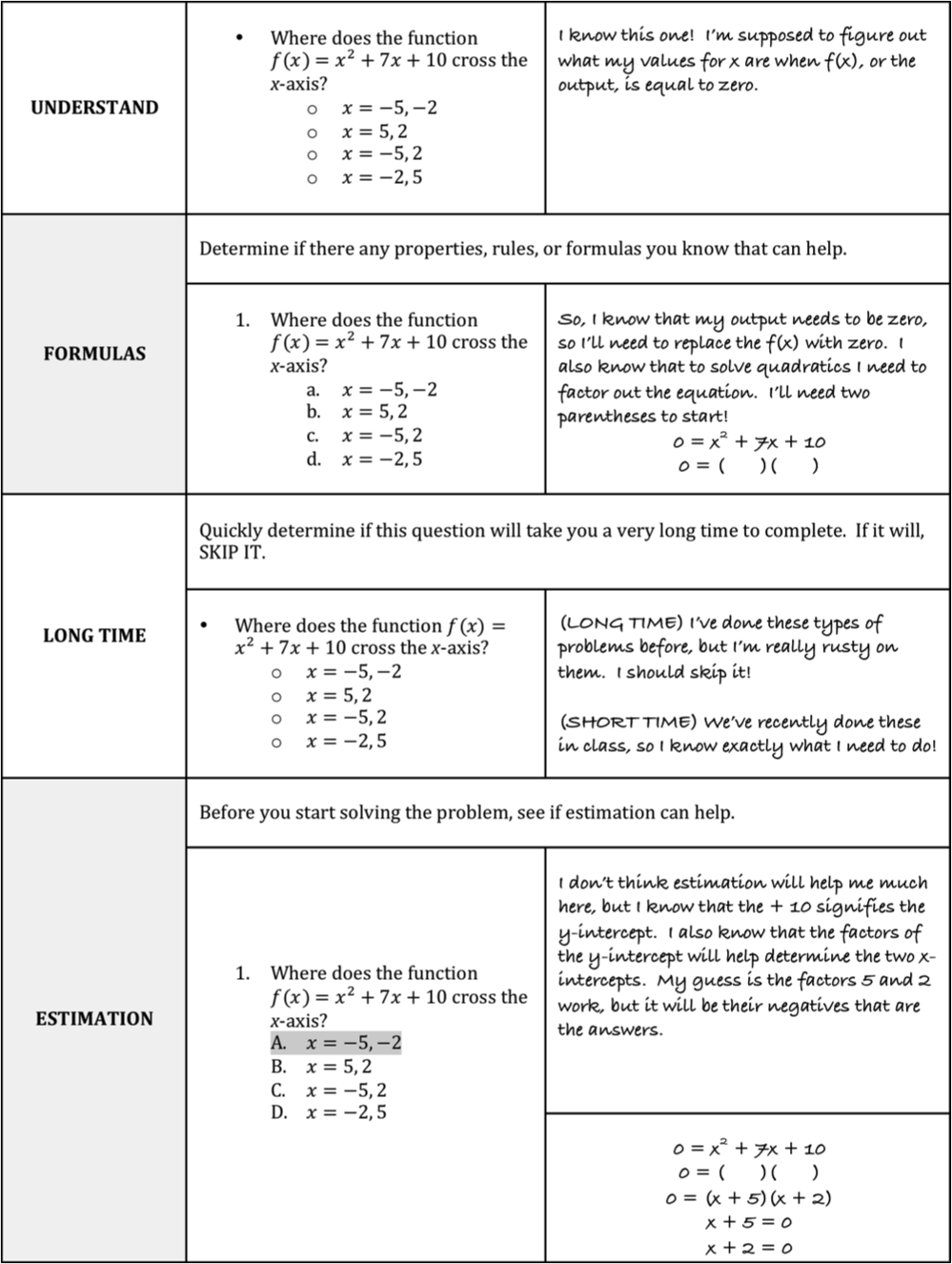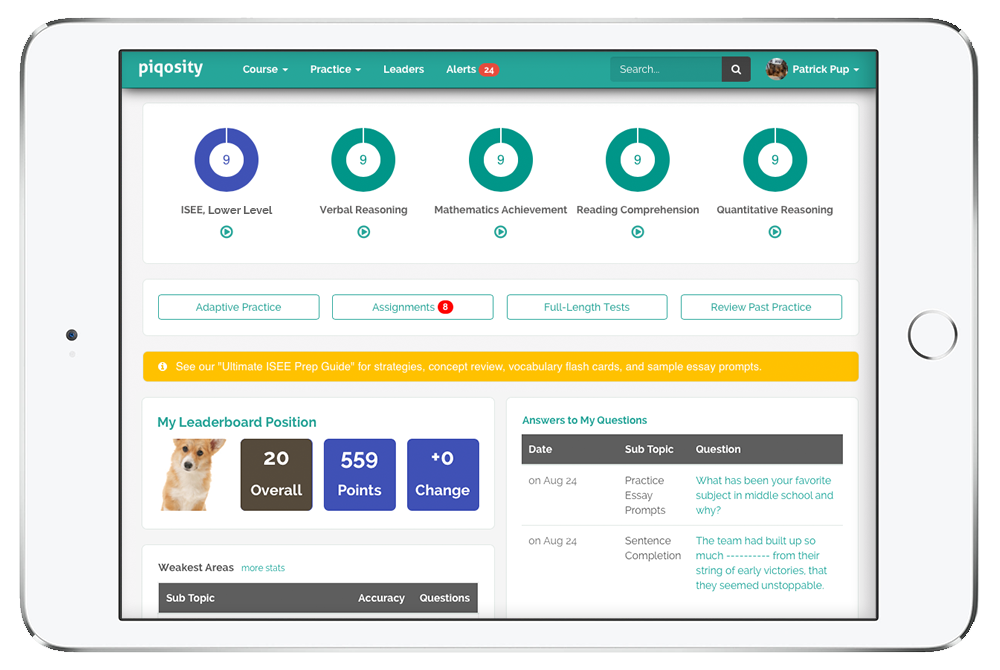# ISEE Mathematics Achievement

### For the Upper Level and Middle Level

The Mathematics Achievement section is the longest part of the ISEE. You will have 40 minutes to answer 47 word problems, and you are not allowed to use a calculator. This section is very similar to Quantitative Reasoning, except that it does not have any quantitative comparison questions.

0th
Section
0
Minutes
0
Questions
0
Seconds/ Question

To learn about what level of math you should know for the ISEE and for quick tutorials on the 48 most tested concepts, click on the button below.

## Word Problems

Each word problem is followed by four answer choices. Choose the answer choice that best answers the word problem. Several of the word problems may be solved without actually doing any math on paper.### General 5-Step Approach

 Step Question Yes No 1 Do I understand the question? Write out the necessary information to the side of the problem. SKIP IT! Circle the question in the test booklet and come back to it if time permits. 2 Do I know which formulas I should use? Quickly write out the necessary formulas to the side of the problem. SKIP IT! Circle the question in the test booklet and come back to it if time permits. 3 Will the question take me a long time to complete? SKIP IT! Circle the question in the test booklet and come back to it if time permits. See if you can solve the problem without performing any math. 4 Can I input values to solve the question? Use simple values such as -1, 0, and 1. If the answer chances, then choose D. Keep in mind all properties of algebraic and geometric concepts. 5 Can I make an educated guess with what I know? Eliminate portions of each value that are the same in both columns. DO NOT leave it blank. Choose the answer that makes the most sense.

For Example:##Full-Length Practice Tests

Piqosity offers 10 full-length practice tests each for the ISEE Lower, Middle, and Upper Levels. Learn more and and take a free diagnostic test at Piqosity.com/ISEE-practice-tests.